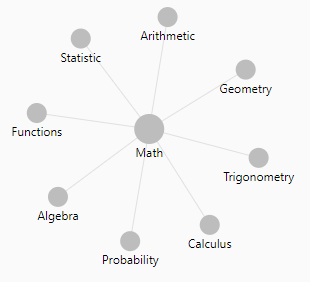# Number - Root function

The root function is the inverse operation of the exponentiation.

$$b^n = a$$

• the base b can be defined back with the root function as:

$$b = \sqrt[n]{a} = a^{\frac{1}{n}}$$

If you need to get the exponent n (power), the operation is not the root function but the logarithm $$n = log_b(a)$$

## Custom

### Square Root

square root is the inverse of the square operation

• If

$$y^2 = x$$

• then

$$y^{\frac{1}{2}} = \sqrty = \sqrt{y} = x$$

Every positive number x has two square roots:

• a positive number known as the principal square root and denoted:
• a negative number:
• $-\sqrt {x}$
• or $-x^{\frac{1}{2}}$

For example: The square roots of 9 are:

• 3 denoted as $\sqrt9$
• and −3 denoted as $-\sqrt9$

## Documentation / Reference

Discover MoreMathematics - Exponentiation (square, cube) - Power

Exponentiation is a binary operation involving two numbers: the base (b) the exponent (n) (or index or power). In text notation or computer language, generally the exponentiation operator...Mathematics - Inverse axiom

/ is inverse of - is inverse of + root is the inverse of exponentiation Functions f and g are functional inverses if: and are defined and are functions.